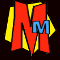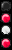# 2043 - The Year That We All Turn Into ELVIS!## Number of Elvises = pe r t

p = the number of Elvis impersonators in 1977.
e = the growth constant which is 2.718281828...
r = the growth factor (we have to work this bit out)
t = the number of years since 1977.

Normally this formula would be applied to the interest you should earn from having money in the bank. P would be the premium (i.e. the money you start with), r would be the interest rate (usually as a decimal) and t would be the number of years you have the money in for.